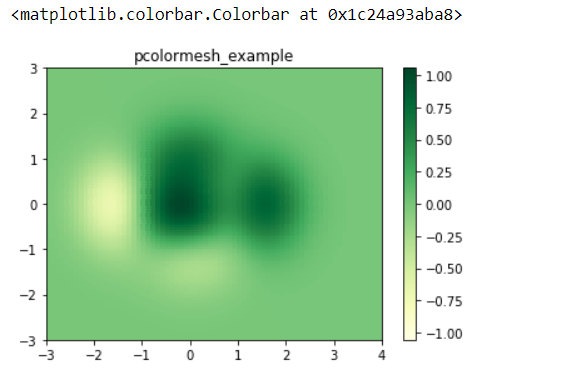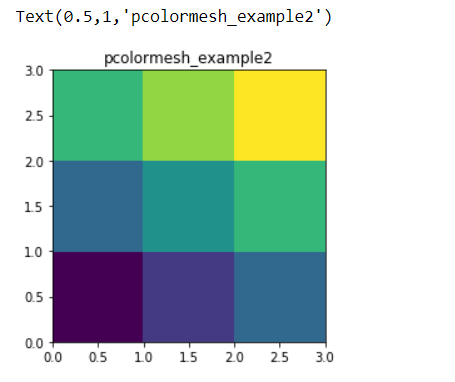# Plot a quadrilateral mesh in Python using Matplotlib

Matplotlib a multiplatform data visualization library built on NumPy arrays, and designed to work with the broader SciPy stack. Matplotlib has the ability to play well with many operating systems and graphics backends as well. matplotlib.pyplot can also be used for MATLAB-like plotting framework.

pcolormesh() function of the pyplot module is used which is similar to pcolor() function, but pcolor returns PolyCollection whereas pcolormesh returns matplotlib.collections.QuadMesh. pcolormesh is much faster and hence can deal with larger arrays.

Syntax : pcolormesh(cmap = [None | Colormap], alpha = [0<=scalar<=1 | None], edgecolors = [None | color | 'face'], shading = ['gouraud' | 'flat'], norm = [None | Normalize], vimax = [scalar | None], vimin = [scalar | None])

Parameters:

1. cmap : It can be None or matplotlib has a number of built-in colormaps accessible via matplotlib.cm.get_cmap
2. alpha : It can be None or alpha value between 0 to 1.
3. edgecolors :
• If its None, edges will not be visible.
• ‘face’ represents the same color as faces.
• color sequence will set a color.
4. shading : It can be either ‘flat’ or ‘gouraud’
5. norm : If its None defaults to normalize().
6. vimax : It can be either None or the scalar value.
7. vimin : It can be either None or the scalar value. ( vimax and vimin are used in conjunction with normalize data)

Example 1 :

 `import` `matplotlib.pyplot as plt ` `import` `numpy as np ` ` `  ` `  `x1, y1 ``=` `0.1``, ``0.05` ` `  `# generate 2-D grids for the ` `# x & y bounds ` `y, x ``=` `np.mgrid[``slice``(``-``3``, ``3` `+` `y1, y1), ``slice``(``-``3``, ``4` `+` `x1, x1)] ` `z ``=` `(``1` `-` `x ``/` `2.` `+` `x ``*``*` `5` `+` `y ``*``*` `3``) ``*` `np.exp(``-``x ``*``*` `2` `-` `y ``*``*` `2``) ` ` `  `# Remove the last value from the ` `# z array as z must be inside x ` `# and y bounds. ` `z ``=` `z[:``-``1``, :``-``1``] ` `z_min, z_max ``=` `-``np.``abs``(z).``max``(), np.``abs``(z).``max``() ` ` `  `plt.subplot() ` ` `  `plt.pcolormesh(x, y, z,  ` `               ``cmap ``=``'YlGn'``,  ` `               ``vmin ``=` `z_min,  ` `               ``vmax ``=` `z_max, ` `               ``edgecolors ``=` `'face'``, ` `               ``shading ``=``'flat'``) ` ` `  `plt.title(``'pcolormesh_example'``) ` ` `  `# set the limits of the plot ` `# to the limits of the data ` `plt.axis([x.``min``(), x.``max``(), y.``min``(), y.``max``()]) ` ` `  `plt.colorbar() ` `plt.show() `

Output :Example 2 :

 `import` `matplotlib.pyplot as plt ` `import` `numpy as np ` ` `  ` `  `x ``=` `np.array([[``0``, ``1``, ``2``, ``3``],  ` `              ``[``0``, ``1``, ``2``, ``3``], ` `              ``[``0``, ``1``, ``2``, ``3``], ` `              ``[``0``, ``1``, ``2``, ``3``]])  ` ` `  `y ``=` `np.array([[``0.0``, ``0.0``, ``0.0``, ``0``], ` `              ``[``1.0``, ``1.0``, ``1.0``, ``1``],  ` `              ``[``2.0``, ``2.0``, ``2.0``, ``2``], ` `              ``[``3``, ``3``, ``3``, ``3``]])  ` ` `  `values ``=` `np.array([[``0``, ``0.5``, ``1``],  ` `                   ``[``1``, ``1.5``, ``2``], ` `                   ``[``2``, ``2.5``, ``3``]]) ` ` `  `fig, ax ``=` `plt.subplots() ` ` `  `ax.pcolormesh(x, y, values) ` `ax.set_aspect(``'equal'``) ` `ax.set_title(``"pcolormesh_example2"``) `

Output :My Personal Notes arrow_drop_upCheck out this Author's contributed articles.

If you like GeeksforGeeks and would like to contribute, you can also write an article using contribute.geeksforgeeks.org or mail your article to contribute@geeksforgeeks.org. See your article appearing on the GeeksforGeeks main page and help other Geeks.

Please Improve this article if you find anything incorrect by clicking on the "Improve Article" button below.

Article Tags :

Be the First to upvote.

Please write to us at contribute@geeksforgeeks.org to report any issue with the above content.